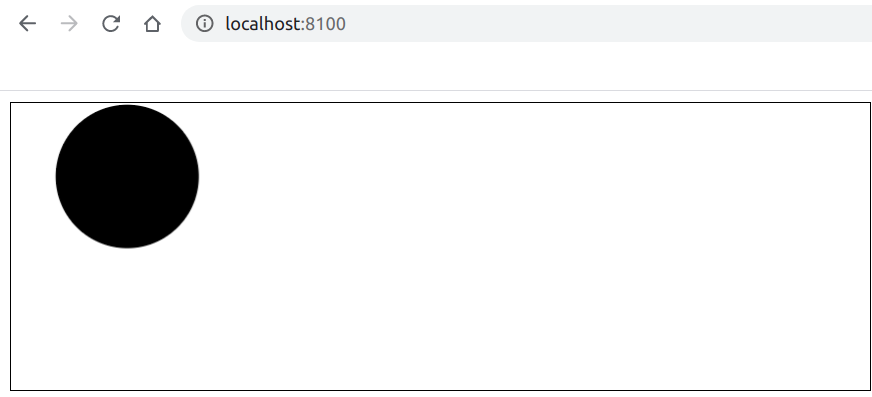# How to set position relative to left of a canvas circle using Fabric.js ?

• Last Updated : 12 May, 2020

In this article, we are going to see how to position relative to the left of a canvas circle using FabricJS. The canvas means the circle is movable and can be stretched according to requirement. Further, the circle can be customized when it comes to initial stroke color, fill color, stroke width, or radius.

Approach: To make it possible, we are going to use a JavaScript library called FabricJS. After importing the library using CDN, we will create a canvas block in the body tag which will contain our circle. After this, we will initialize instances of Canvas and Circle provided by FabricJS and position relative to the left of the circle using left property and render the Circle on the Canvas as given in the example below.

Syntax:

```fabric.Circle({
left: number
}); ```

Parameters: This function accepts two parameters as mentioned above and described below:

 ```<``html``>`` ` `<``head``>``    ``<``title``> ``        ``How to position relative to left of``        ``a canvas circle using FabricJS?``    ```` ` `    ````    ``<``script` `src``=``"https://cdnjs.cloudflare.com/ajax/libs/fabric.js/3.6.2/fabric.min.js"``>``    `````` ` `<``body``>``    ``<``canvas` `id``=``"canvas"` `width``=``"600"` `height``=``"200"` `        ``style``=``"border:1px solid #000000"``>``    ```` ` `    ``<``script``>`` ` `        ``// Initiate a Canvas instance``        ``var canvas = new fabric.Canvas("canvas");`` ` `        ``// Initiate a Circle instance``        ``var circle = new fabric.Circle({``            ``radius: 50,``            ``left: 30``        ``});`` ` `        ``// Render the circle in canvas``        ``canvas.add(circle);``    `````` ` ``